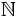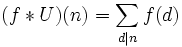# All ones function

This article defines an arithmetic function or number-theoretic function: a function from the natural numbers to a ring (usually, the ring of integers, rational numbers, real numbers, or complex numbers).
View a complete list of arithmetic functions

## Definition

Letbe a commutative unital ring. The all ones function from the natural numberstois the function that sends every natural number to the identity elementof.

The all ones function is typically denoted by the letter.

## Relation with other arithmetic functions

• The inverse of this function with respect to the Dirichlet product is the Mobius function.
• The square of this function with respect to the Dirichlet product is the divisor count function.
• Taking the Dirichlet product of a functionwith this function is equivalent to summing upover all the positive divisors:.